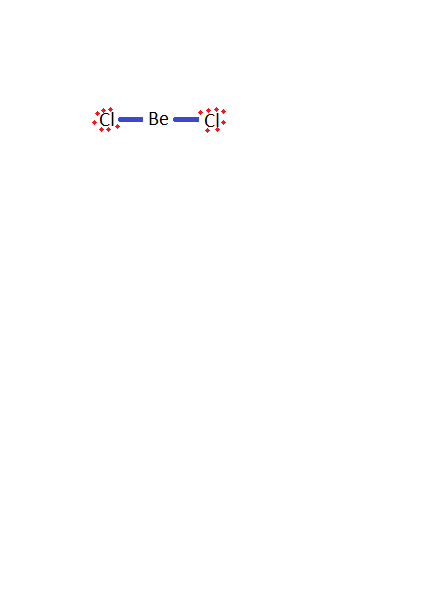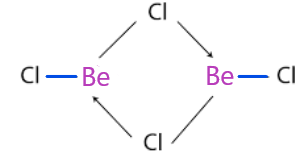QuestionAnswers

# How many 3C - 4${{\text{e}}^{-}}$ bonds are present in the dimer of $\text{BeC}{{\text{l}}_{2}}$?

Hint: 3C-4${{\text{e}}^{-}}$ bonds stands for three centre - 4 electron bonds. Coordination bonds are those bonds which are made after the sharing of lone pair electrons. 3C-4${{\text{e}}^{-}}$ bond means that in between 3 atoms total 4 electrons are shared which do not participate in the reaction.-So, beryllium chloride dimerises to form $\text{B}{{\text{e}}_{2}}\text{C}{{\text{l}}_{4}}$ because it is unstable due to incomplete octet present in the boron and the structure of it is:Therefore, total 2, 3C - 4${{\text{e}}^{-}}$ bonds in the structure of beryllium chloride.
Note: Electron deficient molecules tend to accept electrons so they can make coordinate bonds easily. Other molecules which can form 3C - 4${{\text{e}}^{-}}$bonds are $\text{Xe}{{\text{F}}_{2}}\text{, A}{{\text{l}}_{2}}\text{C}{{\text{l}}_{6}}\text{, HF, etc}$. Electron rich molecules are those species which have an extra pair of electrons to make the bond with electron deficient molecules.